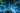# Determinism in ML modelling

Improve determinism in machine learning pipelines by seeding pseudorandom number generators.

# Determinism in ML modelling

Training models in Caffe, PyTorch, R or TensorFlow can quickly devolve into an organizational challenge as teams and models grow. Specifying the seeds to pseudorandom number generators aids in obtaining more control over "randomness" within such setups.

Grotesquely simplified, repeatability (Wikipedia) speaks to the variance between the results of the exact same test executed in succession within a given lab whereas reproducibility (Wikipedia) speaks to the variance between the results of the test when executed in accordance to the same methodology but perhaps by different groups in different labs using slightly different setups.

Although, one aims to design models that generalize well enough to some measure of input variance, one may still want the flexibility in being able to rerun a trial and yield similar results to previous trials even if it is just convenient for analysis or debugging purposes.To this end, we have started to specify the seeds to bootstrap pseudorandom number generators, abbreviated as PRNG's, since nearly all learning methods utilize such generators to initiate some parameters. Observe how we've set the seed values to 42 in the following snippet based on one of the examples from the TensorFlow tutorials.

import tensorflow as tf

# PREPROCESSING STAGE
import numpy as np

# Use seeds to keep the production of the datasets deterministic
np.random.seed(42)
data = np.random.random((2000, 32))

np.random.seed(42)
labels = np.random.random((2000, 10))

training_data = data[:1000]
training_labels = labels[:1000]

validation_data = data[1000:]
validation_labels = labels[1000:]

training_tuple = (training_data, training_labels)
validation_tuple = (validation_data, validation_labels)

training_set = tf.data.Dataset.from_tensor_slices(training_tuple)
validation_set = tf.data.Dataset.from_tensor_slices(validation_tuple)

# TRAINING STAGE
tf.compat.v1.random.set_random_seed(1234)
model = tf.keras.models.Sequential([
tf.keras.layers.Dense(64, activation='relu'),
tf.keras.layers.Dense(64, activation='relu'),
tf.keras.layers.Dense(10, activation='softmax')
])

model.compile(
optimizer=tf.train.AdamOptimizer(0.001),
loss='categorical_crossentropy',
metrics=['accuracy']
)

# Set a random seed to keep the fitting of the model deterministic
tf.set_random_seed(42)
model.fit(
training_data, training_labels,
epochs=10,
batch_size=32,
validation_data=(validation_data, validation_labels)
)

This is just one measure which we introduced in our workflow to allow us to reproduce results or control and monitor input changes to existing models in a more controlled manner.

In our experience with Caffe, PyTorch, R and TensorFlow we've always been able to find methods/functions to provide us some control over PRNG's.

We use CI variables to keep tabs on the seeds fed into the different jobs within our defined pipelines. The magic number 42 in the presented snippet would therefore be substituted for values from environment variables or configuration files. Our CI setup, accepts or generates the seeds which are subsequently stored to be referenced at reruns. This requires just a bit of work in terms of tooling on our end in order to manage seeds, but it allows us to rerun previous jobs with a high rate of confidence that the output will yield similar results. With those factors locked down, we've often found it easier to debug the models we've been crafting or to reproduce the results we encounter while walking down the audit trail.

In practice, we partition data into the desired number of test, validation and training datasets in separate preprocessing pipelines. Subsequently, we provide this data as-is to the workers in the training and validations pipelines. In all stages, one may benefit from specifying or at least recording seeds, although one should question the merit of running jobs with previously used seeds if dependent variables (e.g.: source code, input data, and hyperparameters, among others) remain unchanged. For every job that depends on seeds (data processing or training), we generate new seeds in order to allow for the variance that such methodologies leverage to converge to some global optima rather than some local optima.

Examine this code on Colaboratory but note that this only yields reproducible results when executed in a newly instantiated runtime. Within your pipelines, this would not be an issue as long as you're instantiating new runtime environments for every invocation e.g.: an ephemeral docker instance where the code runs. Running this code multiple times within the same machine will result to outputs that may vary although deterministically over time.